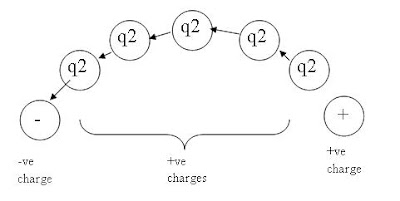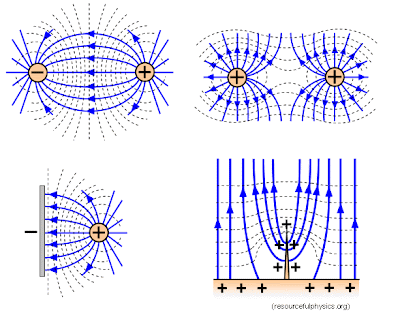## Sunday, April 12, 2009

### 2.1 Coulomb's Law

Previous sections:
1. Introduction
2. Electrostatic

2.1 Coulomb's Law

Most scientific theories up to the late 19th century are based on Newtonian forces. So it's not unusual that the investigation of electrostatic should also begin with the force that is acting on an electric charge.

Let's begin our little experiment by isolating a single sphere of negative charge.

***********************************************
We like to assume these charges are sphere-like because we assume that they exert a force in all directions. But how does a charge actually looks like is altogether a very complex question to answer. Also, beware of the term 'single charge'. What is 'single'? Is there such thing as one charge? Without units, these terms become ambiguous. But for the moment, let's assume that there is such a thing called 'unit charge'.
***********************************************

Now, we all know from A-level physics that like charges repel and opposite charges attract. So if we then place a positive charge near to it, then both will experience a force that pulls them together. But for the moment, let's assume that the first charge, q1, is fixed in spaced and concentrate only on what happens to the second charge, q2.

To visualise this, we represent the direction of the force with an arrow. We repeat the experiment a number of times, each time with q2 placed in a slightly different position such that the end of the arrow coincides with the beginning of another arrow, this is what we might get.Imagine if the experiments are repeated so many times and that the arrows become very small, it then forms a continuous straight line. If we place q2 in a space with two charges of opposite polarity, it becomes clearer as to what we are trying to achieve here.These lines that we traced by repeating the experiment numerous time are called lines of action or more commonly known as field lines. They represent the direction which the force will be acting. In other words, if a charge is placed on a particular line, the force will act on it such that it will move along the line.

***********************************************
Generally, fields can be scalar or vector. In this case, electric field refers to the action of the force that pulls or pushes a charge. Force is a vector. Therefore, the electric field is also a vector.
***********************************************So field lines are really just imaginary lines that scientists cooked up to explain what they observe in the experiments. They do not physically exist! The field lines are there just to help us to visualise. One can imagine that by placing a charge in space, field lines will emanate from it and exert forces on other nearby charges in the direction of the field lines.

Since electric fields are just some sort of forces, we can now choose to define it as the force per unit charge. We could have define it any other way (as long as it tells us something about the force), but scientists have found that this definition is the most useful (and they were right!) so we will just follow. But when we say force per unit charge, which charge are we referring to? q1 or q2? This depends on which charge the force is acting on, i.e. in this case it is q2. In other words, electric fields will tell you how much force a charge (q2) will be experiencing when it is placed in an electric field. We now have

E=F/q2

where E is the electric field (Newton per Coulomb), F is the force (Newtons) and q is the charge (Coulombs).

***********************************************
The reader may notice that we have not define the unit of Coulomb or how much is a single charge. We will come that later.
***********************************************

We now turn our attention back to the electric field. What causes it? The answer is obviously q1. Since the field lines are 'generated' by the presence of the charge, q1, it is natural to think that if q1 is 'stronger' or 'has more quantity of charges', then the field that emanates from it will be 'stronger' and the forces of attraction or repel will also be stronger. Just like in gravity, the bigger the mass the bigger the pull. And so it is reasonable to say that the electric field, E, is proportional to q1, i.e.

E = k . q1

Charles-Augustin de Coulomb, a French physicist, postulated that the force between two electric charges, just like gravity, has the inverse square law (i.e. proportional to 1/r^2) and experiments confirmed that his postulate was indeed correct. On this postulate, we can now further improve our above equation to

E = k . q1 / (4*π*r^2).

Hang on a minute! I said "inverse square law". I did not mention anything about the π. So where does the 4*π*r^2 comes from? Now, imagine a pulse of energy emanating from a single point. As time progresses, the energy spreads out in a growing sphere. The energy resides only on the surface of the sphere. Then, the energy density could be easily calculated as

Energy / Surface of the sphere = Energy / 4*π*r^2

Electric fields are not energy. But scientists found that other than energy, many of their observations in nature also obey this law. So, it was natural for Coulomb to assume electric fields behave like this as well. So, I emphasize again, that the inverse r^2 dependence is merely a postulate, or just a guess, if you will. This postulate was confirmed by experiments in Coulomb's days, and also with high-precision experiments of more recent days up to about 10^-12 cm and possibly even smaller. Unless an experiment can show otherwise, this postulate will hold.

A quick recap of what we have know so far,
F = q2 . E
E = k . q1 / (4*π*r^2)

which gives us,
F = k . q1 . q2 / (4*π*r^2)

Since at the point of this experiment in history, the value of k and unit charge (for q1 and q2) have yet to be decided, it is at our own discretion to choose a suitable value for them, as long as it matches with the observations in the experiments. But, as always, the scientists whom discovered the phenomenon have done this for us. For historical reasons, the value for k = 1/ε_0 and the unit for charge is Coulomb, as some of you may already know.

*************************************************
ε_0 is known as the permittivity of free space and the value is approximately 8.854x10^-12 and the unit is Farad per meter.

While 1 Coulomb is defined this way: If at each end of a meter is 1 Coulomb of charge, each charge will experience a force equivalent to 9x10^9N.
*************************************************

Plugging this in, we finally have the equation for the famous Coulomb's Law,

F = q1 . q2 / (4*π*ε*r^2)

and the corresponding electric field is

E = q1 / (4*π*ε*r^2)

This equation gives only the magnitude. Since electric field and force is a vector, we denote a_r as the unit vector pointing in the direction of where the force is acting. So, finally we get

F = a_r . q1 . q2 / (4*π*ε*r^2)
E = a_r . q1 / (4*π*ε*r^2)

It is now worthwhile to note that in our example above, it was assumed q2 did not 'generate' any field of its own when placed next to q1. In reality, this is not true. q1 and q2 both 'generate' their own fields and by placing q2 next to q1, their field lines superimpose to form new field lines. It is this new field lines that will determine the forces and how these charges pull or push each other. As you can imagine by now, things get messy very quickly when the number of charges that we consider becomes more than 2.

#### 1 comment:Anonymous said...

Just discover your blog. Good job man. I found them very useful. Nice presentation